Famous People From India
All the inventions and discoveries in the field of mathematics would have meant nothing had it not been for the introduction of zero to mathematics. And do you know who introduced zero to maths? Well, it was an Indian mathematician and genius Brahmagupta. Born in 598 AD, he not just made known the concept of zero in arithmetic but also explained how to find cube and cube root of an integer. But is that all what Indian mathematicians gave to the field of maths? Modestly no! Aryabhatta, one of the earliest Indian mathematicians, first announced to the world that the earth was spherical and that it revolved around the sun. Furthermore, he was the first to state explicitly that a year comprises of 365 days and the first to work on place value system using letters to signify numbers. Other prominent Indian mathematicians include Srinivasa Ramanujan, Satyendra Nath Bose, Bhaskara, and so on. Interestingly, prominent Indian mathematicians have spanned across many centuries, dating back from the Indus Valley civilization and Vedas to modern times. Apart from legendary mathematicians, Indian soil has been home to some of the prodigious mathematic talent such as Shakuntala Devi. Fondly called human computer, she was said to have possessed unbelievable mental calculating skills. Check this section to know in details about famous Indian mathematicians, their life and their contribution to maths.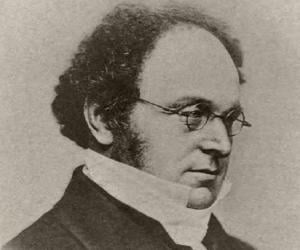Augustus De Morgan
27 June 1806
British mathematicianS. R. Ranganathan
12 August 1892
Mathematician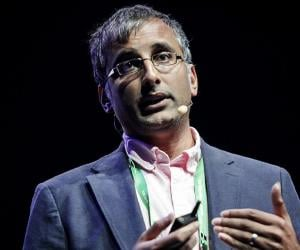Akshay Venkatesh
21 November 1981
Mathematician, University teacherBhāskara I
Mathematician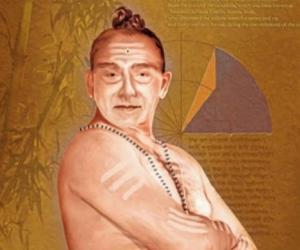Madhava of Sangamagrama
mathematician, astronomer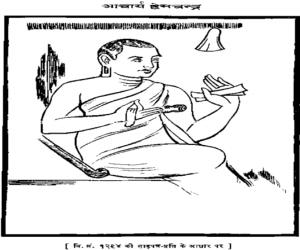Hemachandra
mathematician, historian, philosopher, writer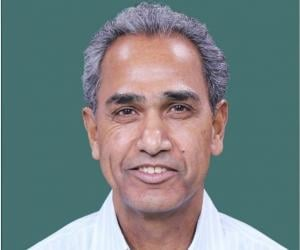Harish-Chandra
11 October 1924
mathematician, physicist, university teacher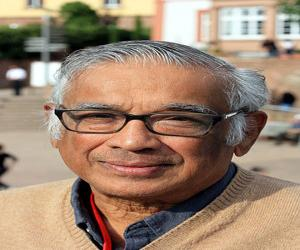S. R. Srinivasa Varadhan
02 January 1940
Mathematician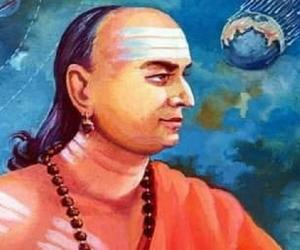Varahamihira
AstronomerManindra Agrawal
20 May 1966
Mathematician, Computer scientist, UniversityNilakantha Somayaji
14 June 1444
mathematician, astronomer, astrologerMahan Mj
05 April 1967
mathematicianDignāga
ScholarRaj Chandra Bose
19 June 1901
Mathematician, Statistician, University teacherRaghunath Dhondo KarveZiauddin AhmadJ. H. C. Whitehead
11 November 1904
MathematicianR. P. ParanjpeS. Ramanan
20 July 1937
mathematicianGanesh Prasad
15 November 1876
MathematicianRamchundraBapudeva Sastri
01 November 1821
ScholarShridharaShripati